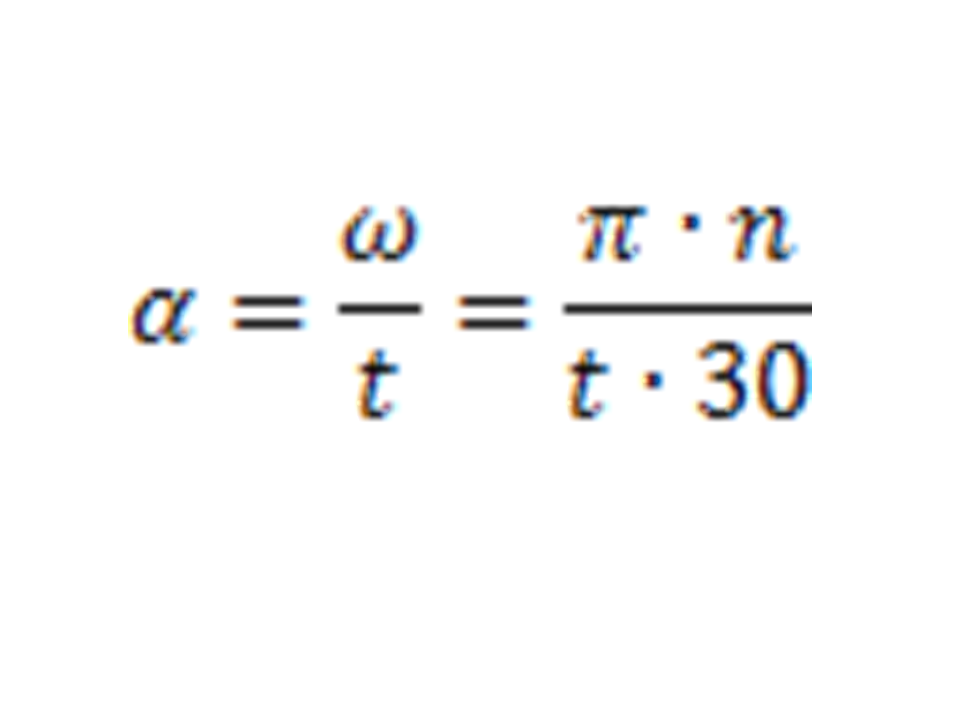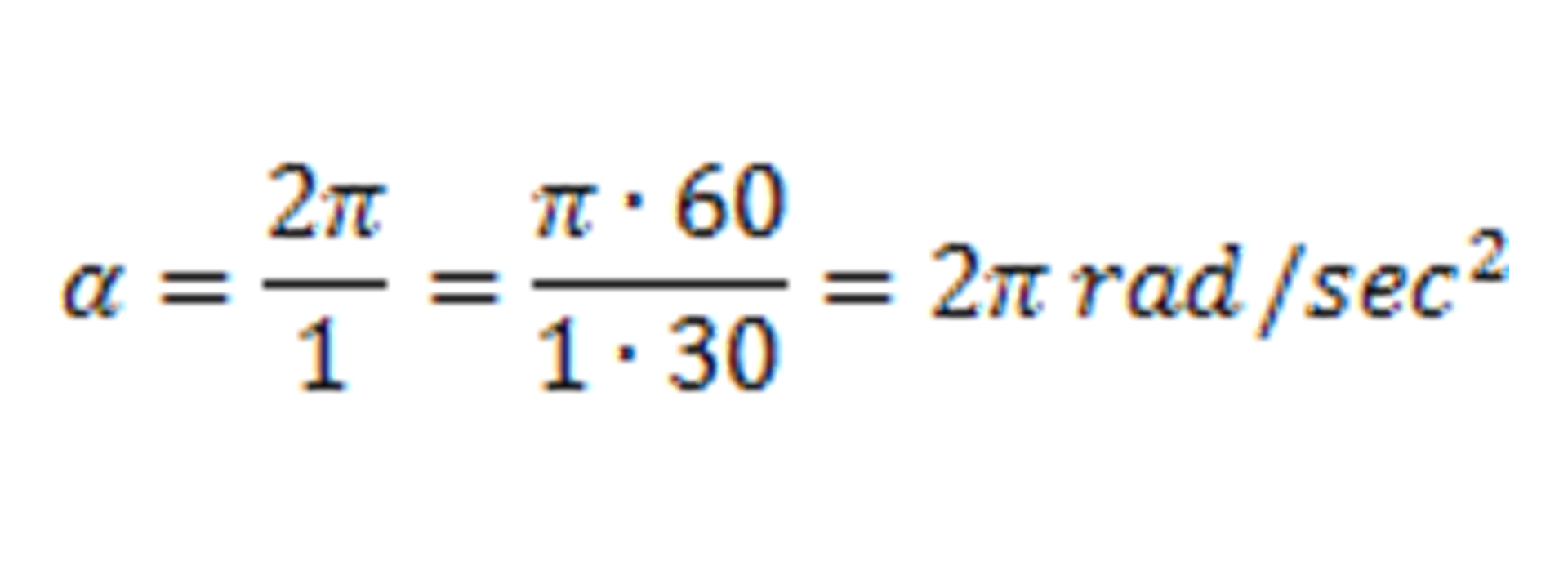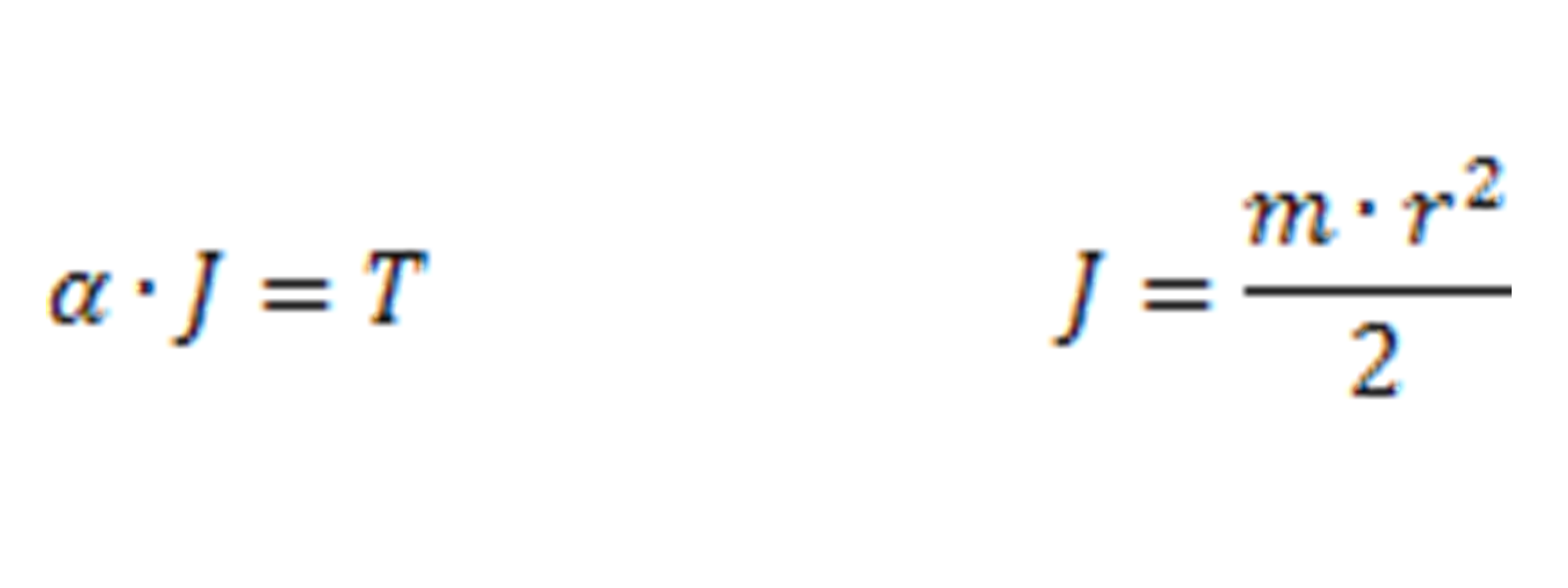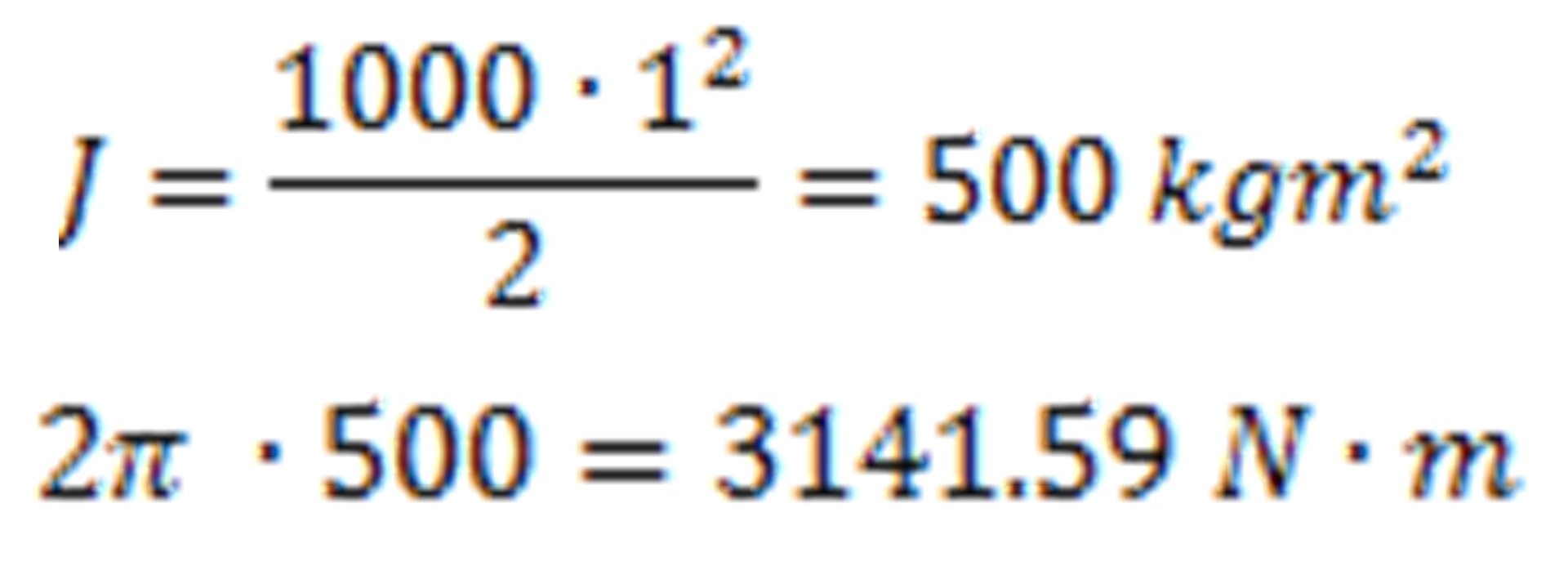This is where you give the visitor a brief introduction to both this blog and your company. Keep the intro pithy and punchy.

# Blog#### Angular Acceleration and Moment of Inertia in Machine Design

As a provider of flexible drive couplings and ball detent safety clutches, we are often asked to provide a bit of assistance in calculating application torques, especially for customers looking to retrofit existing equipment.  In order to aid in the process of estimating torques, we'll review one of the basic calculations used to estimate the torque required to accelerate a rotating mass to a certain speed over a given time.

Angular acceleration (α) can be defined as angular velocity (ω) divided by acceleration time (t). Alternatively, pi (π) multiplied by drive speed (n) divided by acceleration time (t) multiplied by 30. This equation yields the standard angular acceleration SI unit of radians per second squared (Rad/sec^2). The equation below defines the rate of change of angular velocity.ω = angular velocity in the standard SI unit of radians per second (Rad/sec), 1 radian = 57.3 degrees

t = acceleration time in seconds

π = 3.1416

n = drive speed in revolutions per minute RPM

In the next example, angular velocity will be calculated for acceleration from 0 to 60 RPM in one second. Note that 2π radians per second = 60 RPM.This calculation is very useful in machine design because angular acceleration multiplied by rotational moment of inertia equals torque.  Keep in mind that the exact moment of inertia can be difficult to calculate based on complex geometries in real drive lines, and other variables such as friction are not considered in the next calculation.  Nonetheless it is still very helpful in approximating torque requirements or establishing baseline minimum values for component sizing purposes.J = moment of inertia in kg∙m2

T= torque in N∙m

N= force in Newtons

kg= mass in kilograms

m= lever arm radius in meters

In the final example below we will use the angular acceleration we found above to calculate torque on a flywheel with a 1 meter radius and 1000 kg mass.As we can see, if a flywheel with a 1 meter radius and 1000 kg mass were to be accelerated to 60 RPM in one second, it would require 3141.59 Newton meters of input torque.

I hope you found this refresher on calculating angular acceleration to be helpful.  If you have questions pertinent to the sizing and application of shaft couplings or safety clutches, feel free to contact our applications engineering department.

applications@rw-america.com